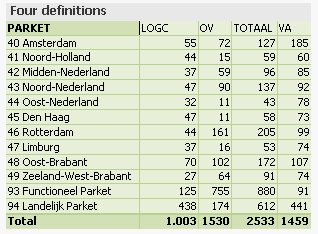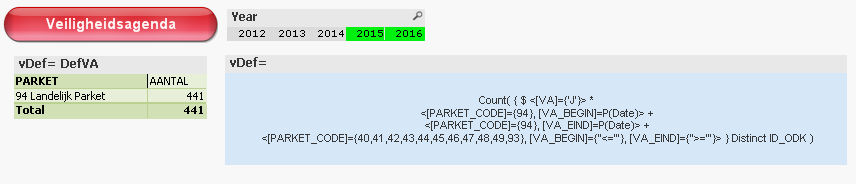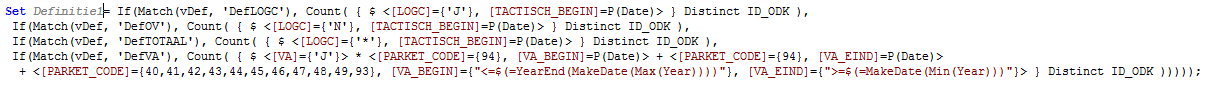# QlikView Creating Analytics

Discussion Board for collaboration related to Creating Analytics for QlikView.

Announcements
IMPORTANT: Upcoming LEF Database Maintenance, Oct. 3rd - SEE DETAILS
cancel
Showing results for
Did you mean:
HighlightedNot applicable

## Expression as a variable with \$-sign expansion

I have a Pivot Table Chart with four different expressions.I want to change this chart in a way that it only shows one expression and I can choose the desired calculation (expression) with a button. Therefore I put the expressions in four variables.

Set DefLOGC=    Count( { \$ <LOGC]={‘J’}, [TACTISCH_BEGIN]=P(Date)> } Distinct ID_ODK );

Set DefOV=         Count( { \$ <LOGC]={‘N’}, [TACTISCH_BEGIN]=P(Date)> } Distinct ID_ODK );

Set DefTOTAAL= Count( { \$ <LOGC]={‘*’}, [TACTISCH_BEGIN]=P(Date)> } Distinct ID_ODK );

Set DefVA=         Count( { \$ <[VA]={‘J’}> *

<[PARKET_CODE]={94}, [VA_BEGIN]=P(Date)> +

<[PARKET_CODE]={94}, [VA_EIND]=P(Date)> +

<[PARKET_CODE]={40,41,42,43,44,45,46,47,48,49,93},

[VA_BEGIN]={‘<=\$(=YearEnd(MakeDate(Max(Year))))’},

[VA_EIND]={‘>=\$(=MakeDate(Min(Year)))’}> }

Distinct ID_ODK );

The button stores the name of the definition (DefLOGC, DefOV, DefTOTAAL or DefVA) in a variable vDef. In the chart I use the expression \$(=\$(vDef)). This works for the variables (expressions)  DefLOGC, DefOV and DefTOTAAL, but not for DefVA.I suppose it has to do with the use of \$-sign expansions in the expression, if stored as a variable. It makes no difference if I put double quotes (“”) around the expressions. I have read discussions and blogs on Qlik Community and elsewhere, but can not find a working solution for this problem. I am running out of ideas. Anyone else?

I am using QlikView Version 11.20 SR10 (64-bit)

1 Solution

Accepted Solutions
HighlightedMVP

Your issue is caused by QV trying to evaluate the dollar sign expansion in the script, which will not work with your expression. You need to prevent QV from doing this, there are a couple of methods:

- load the variables from a table source

- replace the \$ with a dummy character and use LET and replace to replace the characters

- use a concatenated string, spearating \$ from ( and thus preventing the DSE

There are a couple of threads that demonstrate all these methods here in the forum (I think the first is also mentioned in the ref manual).

For example, the last method might look like

LET DefVA=         'Count( { \$ <[VA]={‘'J'’}> *

<[PARKET_CODE]={94}, [VA_BEGIN]=P(Date)> +

<[PARKET_CODE]={94}, [VA_EIND]=P(Date)> +

<[PARKET_CODE]={40,41,42,43,44,45,46,47,48,49,93},

[VA_BEGIN]={'‘<=\$' & '(=YearEnd(MakeDate(Max(Year))))'’},

[VA_EIND]={‘'>=\$' & '(=MakeDate(Min(Year)))’'}> }

Distinct ID_ODK )';

Note also that I replaced all existing single quotes with two single quotes to escape the quote.

Stop Dollar Sign Expansion in the script (Escape Character ??? )

13 Replies
HighlightedMVP

Can you try this -> \$(=vDef)

HighlightedMVP

Alternatively why don't you use a variable to conditionally show hide 4 expression using the conditional expression on the Expression tab?

HighlightedNot applicable

If I use \$(=vDef) instead of \$(=\$(vDef)) it is showing the text of the expression and not the calculated result.HighlightedNot applicable

Yes, I can conditionally show/hide the expressions, depending on vDef. However, I don't want to do that for every expression in every chart or table (and there are a lot). Using an if-statement in the expression, works for DefLOGC, DefOV, DefTOTAAL but not for DefAV.HighlightedMVP

Keeping it \$(=vDef) what do you see when you toggle to DefAV?

HighlightedNot applicable

Sorry, it should be DefVA, not DefAV. I changed it in the expression and now it works. Next step is to put this expression in a variable. I will try that tomorrow. Thank you so far.

HighlightedMVP

Your issue is caused by QV trying to evaluate the dollar sign expansion in the script, which will not work with your expression. You need to prevent QV from doing this, there are a couple of methods:

- load the variables from a table source

- replace the \$ with a dummy character and use LET and replace to replace the characters

- use a concatenated string, spearating \$ from ( and thus preventing the DSE

There are a couple of threads that demonstrate all these methods here in the forum (I think the first is also mentioned in the ref manual).

For example, the last method might look like

LET DefVA=         'Count( { \$ <[VA]={‘'J'’}> *

<[PARKET_CODE]={94}, [VA_BEGIN]=P(Date)> +

<[PARKET_CODE]={94}, [VA_EIND]=P(Date)> +

<[PARKET_CODE]={40,41,42,43,44,45,46,47,48,49,93},

[VA_BEGIN]={'‘<=\$' & '(=YearEnd(MakeDate(Max(Year))))'’},

[VA_EIND]={‘'>=\$' & '(=MakeDate(Min(Year)))’'}> }

Distinct ID_ODK )';

Note also that I replaced all existing single quotes with two single quotes to escape the quote.

Stop Dollar Sign Expansion in the script (Escape Character ??? )

HighlightedNot applicable

With the if-expression stored in a variable "Definitie1" and \$(Definitie1) as expression in the chart, it is not working as it should. See also the reaction of swuehl.Did I understand you wrong?

HighlightedMVP

How do your dollar sign expansions get evaluated in the script within Definitie1 variable?